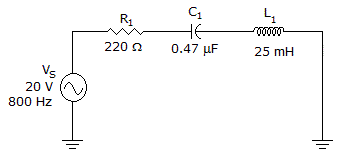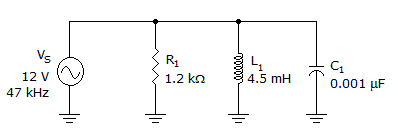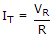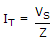# Electronics - RLC Circuits and Resonance

### Exercise :: RLC Circuits and Resonance - General Questions

16.

When a full band of frequencies is allowed to pass through a filter circuit to the output, the resonant circuit is called a:

 A. low-pass filter B. high-pass filter C. band-stop filter D. bandpass filter

Answer: Option D

Explanation:

No answer description available for this question. Let us discuss.

17.

At resonance, the term bandwidth includes all frequencies that allow what percentage of maximum current to flow?

 A. 50 B. 62.3 C. 70.7 D. 95.3

Answer: Option C

Explanation:

No answer description available for this question. Let us discuss.

18.What is the voltage across the capacitor in the given circuit?

 A. 6.8 V B. 11.9 V C. 16.1 V D. 22.9 V

Answer: Option D

Explanation:

No answer description available for this question. Let us discuss.

19.What is the impedance of the circuit in the given circuit?

 A. 1.05 kB. 1.33 kC. 2.19 kD. 3.39 kAnswer: Option A

Explanation:

No answer description available for this question. Let us discuss.

20.

In a series RLC circuit the current can be found using:

 A.B.C. both of the above D. none of the above

Answer: Option C

Explanation:

No answer description available for this question. Let us discuss.

#### Current Affairs 2021

Interview Questions and Answers